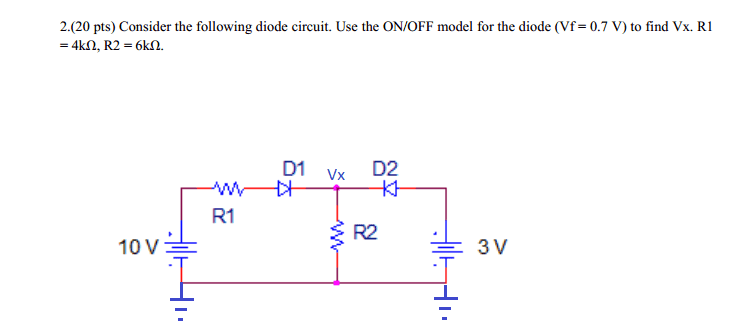# Diode Circuit ON/OFF Model Problem

• Engineering

## Homework Statement## Homework Equations

When Diode is on Vd=0.7 V and Id=0
When Diode is off Vd<0.7 and Id>0

## The Attempt at a Solution

I've been working on this problem for hours now but I just can't logic it out properly. I know the right model is supposed to be D1 ON and D2 OFF, but I can't figure out why or what the value of Vx is. Any help would be greatly appreciated.

NascentOxygen
Staff Emeritus
Hi Shaunzio. You need to consider all possibilities, taking one at a time.

Case (1). D1 is forward biased, D2 is forward biased.
With D2 forward biased, what value does that make Vx?
With that value for Vx, how much current is going through R2, and in what direction?
And so on ...... until you are satisfied you have uniquely determined all circuit parameters.

Case (2). .....

CWatters
Homework Helper
Gold Member
I think there is a slightly shorter method in this case...

I'd start by looking at the case with D2 ON. For D2 to be ON the voltage Vx must be < 3V-0.7 - 2.3V

Try assuming Vx=2.3 V and calculate the current that would flow through R1. That current must also be flowing through R2 (it can't flow the wrong way through D2) so it sets a minimium voltage for Vx. If that's greater than 2.3V then the assumption that D2 is ON is incorrect.

If V2 is off the problem simplifies greatly.